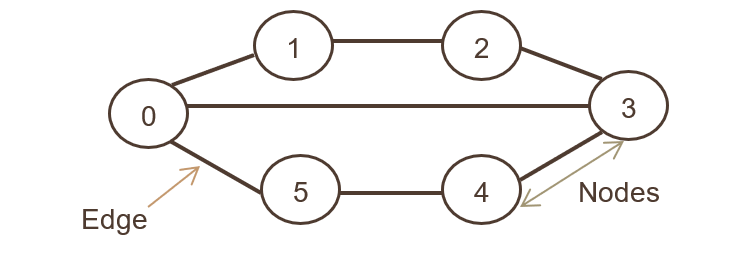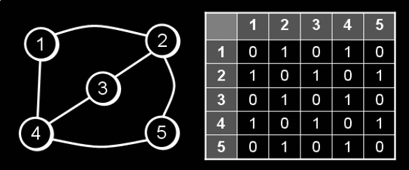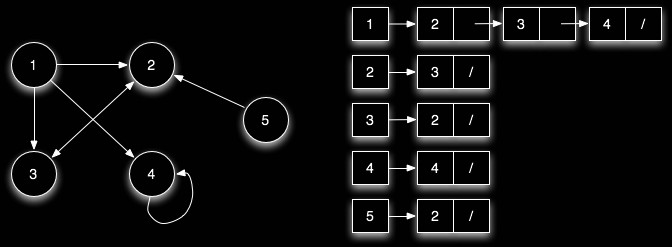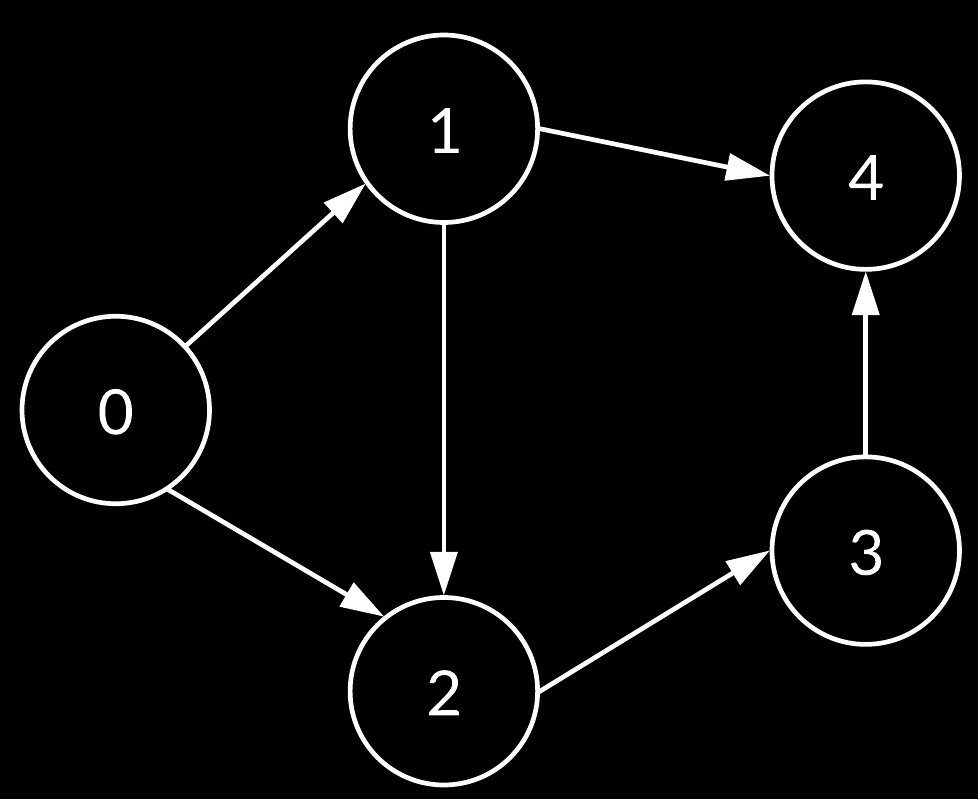# Implementation of Graph in Java

## Introduction

As newbie programmers, almost all of us had thoughts that are these data structures like Arrays, Linked Lists, Trees even used for solving real-world problems, or they are just for cracking the programming interviews? The answer is we use a data structure every day when while fetching the best route to a place using Google Maps, Facebook uses one for the popular "People you may know" feature. If you are guessing this data structure to be a graph, then you are right.

A graph represents data in a non-linear structure consisting of nodes or vertices and edges or paths. This blog will discuss the implementation of Graph in Java with full details. Before moving on to the implementation of Graph, it's necessary to quickly revise Graphs.

## A Brief Introduction to Graphs

A graph is a non-linear data structure that consists of nodes and edges. Formally, a graph can be defined as an ordered set G(V, E) where V represents the vertices and E represents the set of edges that are used to connect these vertices.In the above graph, the set of nodes are {0, 1, 2, 3, 4, 5} and the set of edges are {01, 12, 23, 34, 45, 05, 03}.

In the Facebook example discussed above, everything is a node, including User, Photos, Events, Pages, Comments, Likes, Video, etc. Anything that has data is a node. Every relationship is an edge from one node to another; whether you post a story, make a new friend, like a page, a new edge is created to represent that relationship.

Graphs may be directed or undirected graphs. A directed graph is a set of vertices/nodes connected by edges with each node having a direction associated with it. Edges are represented by arrows pointing to the direction in which the graph can be traversed. If there is an edge between vertex 0 and 1 with an arrow pointing towards 1, then the graph can be traversed from 0 to 1 and not from 1 to 0. In contrast, an undirected graph can be traversed in either direction. The edges are bidirectional. If there is an edge between vertex 0 and 1, then the graph can be traversed from 0 to 1 and from 1 to 0.

Some graphs have weights associated with edges between two vertices. These graphs are called Weighted Graphs.

Implementation of Graph can be done by using either an adjacency list or an adjacency matrix. Each of the two representations has its pros and cons; the choice of a particular graph representation depends on the requirements.

## Implementation of Graph - Using Adjacency Matrix

A graph can be represented using an adjacency Matrix. An adjacency matrix is a square matrix of size V * V, where V is the number of vertices in the graph. The values in the matrix show whether a given pair of nodes are adjacent to each other in the graph structure or not. In the adjacency matrix, 1 represents an edge from node X to node Y, while 0 represents no edge from X to Y.For an undirected graph, the adjacency matrix is always symmetric. For a weighted graph, instead of 0 and 1, the value of weights w is used to indicate that there is an edge from I to j.

Before moving to the code, let us look at the adjacency matrix of directed, undirected and weighted graphs.

### Algorithm

• Make a matrix of size V*V where V is the number of vertices in the graph.
• A new vertex in the graph is added by connecting it with other vertices, and an edge is thus formed. To indicate that there is an edge from vertex i to vertex j, mark matrix[i][j] = 1 and if there is no edge then mark matrix[i][j] = 0.

### Implementation

The below program is used to implement an undirected graph using Adjacency Matrix representation.

// A variable to store the number of vertices in the graph

int vertexCount;

// A variable to store the adjacency matrix of the graph

int matrix[][];

// Constructor to create an adjacency matrix

// of dimensions vertexCount*vertexCount

this.vertexCount = vertexCount;

matrix = new int[vertexCount][vertexCount];

/*

* The matrix[][] for vertexCount = 4 will look like this upon initialization

* '-' indicates 0

* [-, -, -, -]

* [-, -, -, -]

* [-, -, -, -]

* [-, -, -, -]

*/

}

// Function to add an edge from source to destination

// i.e. there is an edge from source to destination

// O(1) time complexity

public void addEdgeInMatrix(int source, int destination) {

matrix[source][destination] = 1;

matrix[destination][source] = 1;

}

// Function to delete an edge from source to destination

// O(1) time complexity

public void deleteEdgeInMatrix(int source, int destination)

{

// Deleting an edge from source to destination

matrix[source][destination] = 0;

// Deleting edge from destination to source

matrix[destination][source] = 0;

}

// Utility function to print a graph

public void printGraph() {

System.out.println("Representation of Graph in the form of Adjacency Matrix: ");

for (int i = 0; i < vertexCount; i++) {

for (int j = 0; j < vertexCount; j++) {

System.out.print(matrix[i][j] + "   ");

}

System.out.println();

}

for (int i = 0; i < vertexCount; i++) {

System.out.print("Vertex " + i + " is connected to: ");

for (int j = 0; j < vertexCount; j++) {

if (matrix[i][j] == 1) {

System.out.print(j + " ");

}

}

System.out.println();

}

}

public static void main(String[] args) {

// 0 ----- 1

// 0 ----- 1

// |

// |

// |

// 4

// 0 ----- 1 ---- 2

// |

// |

// |

// 4

// 0 ----->1 ---> 2

// |       |

// |       |

// |       |

// 4       3

// 0 ----->1 ---> 2

// |     / |

// |    /  |

// |   /   |

// 4  /    3

// 0 ----->1----2

// |    / |   /

// |   /  |  /

// |  /   | /

// 4       3

// 0 ----->1----2

// |    / |   /

// |   /  |  /

// |  /   | /

// 4  ----3

System.out.println("\nDeleting an edge from 3 to 4");

}

}

The output of the above program is:

The time complexity for adding an edge in O(1).

The space complexity is O(V^2), where V is the number of vertices in the graph.

Note that in the case of a weighted graph instead of 0 and 1, respective weights will be added in the adjacency matrix.

1. Insertion and deletion of an edge can be done in O(1) time complexity.
2. We can determine if two edges are adjacent to each other in constant time.

1. Using adjacency Matrix consumes a lot of memory of the order of V^2. In the case of graphs with few edges, this leads to a wastage of memory.
2. Traversal of a graph requires O(V^2) time complexity in case of adjacency matrix representation.

## Implementation of Graph - Using Adjacency List

A graph can also be represented in the form of an adjacency list. An adjacency list represents a graph as an array of linked lists, wherein the index of the array represents a vertex and each element of the linked list represents other vertices that form an edge with the vertex.

Consider the following example wherein there is a directed graph of 5 nodes. The graph along with its adjacency list representation is shown in the below figure

##An array represents each node. A linked list is linked to each node, which represents the link of one node to another node, note that the node numbers are not in any specific order, though it's convenient to list the nodes in ascending order as shown in the below adjacency list representation.

In implementing a Graph representation of Graphs using Adjacency List, we will use an ArrayList of ArrayList. ArrayList is a class of Collection Framework; you may read more about it here.

### Implementation

// Adjacency List representation in Java

import java.util.*;

int vertexCount;

// Adding an edge from source to destination

// Adding an edge from destination to source

}

// A utility function to delete an edge from source index of outer arraylist to

// destination index of inner array list.

static void deleteEdge(ArrayList<ArrayList<Integer>> adj, int source, int destination) {

}

System.out.println("Adjacency List representation of Graph: ");

for (int i = 0; i < adj.size(); i++) {

System.out.println("Adjacency List of vertex " + i);

System.out.print(i + " -> ");

for (int j = 0; j < adj.get(i).size(); j++)

System.out.println();

}

}

public static void main(String[] args) {

// Basically we are forming a graph with vertexCount vertices

// To do that an ArrayList of ArrayLists is created and each outer

// ArrayList will contain vertexCount elements. Each vertex will have

// an ArrayList of vertices that are adjacent to it.

int vertexCount = 5;

for (int i = 0; i < vertexCount; i++)

// Add edges to the graph

// Adding an edge from 0 to 1

// Adding an edge from 0 to 4

// Adding an edge from 1 to 2

// Adding an edge from 1 to 3

// Adding an edge from 2 to 3

// Adding an edge from 3 to 4

System.out.println("After removing an edge from graph");

}

}

The output of the above program is:

The time complexity of the get() and add() method of ArrayList is O(1). So the time complexity of the above program is O(1)

The space complexity of the above program is O(V + E) where V is the number of vertices and E is the number of edges formed by vertices. The space complexity will be O(V^2) in the worst case of a complete graph.

Q1) What are Graphs in Data Structure?

Ans 1) A graph is a non-linear data structure that consists of nodes and edges. Formally, a graph can be defined as an ordered set G(V, E) where V represents the vertices and E represents the edges used to connect these vertices.

Q2) How to represent a graph using an adjacency list?

Ans 2) A graph is represented using an adjacency list as shown below:Q3) How to represent a graph apart from the adjacency list and an adjacency matrix?

Ans 3) An edge list can also be used from the graph representation using the adjacency list and an adjacency matrix. An edge list is just a list or array of edges; to represent an edge, we have an array of two vertex numbers of the vertices that the edges are incident on.The edge list of the above graph is [0, 1], [0, 2], [1, 2], [1, 4], [2, 3], [3, 4]]

## Key Takeaways

Graphs are one of the most important data structures from an interview perspective in product-based companies. This article discusses the implementation of Graph in Java. With this done you must practice more and more questions related to Graphs on Codestudio.

You must check out Codestudio to upskill yourself, Codestudio is an excellent platform for curated guided paths, to practice interview problems, and to read blogs curated by experts. Remember Practice is the Key !!